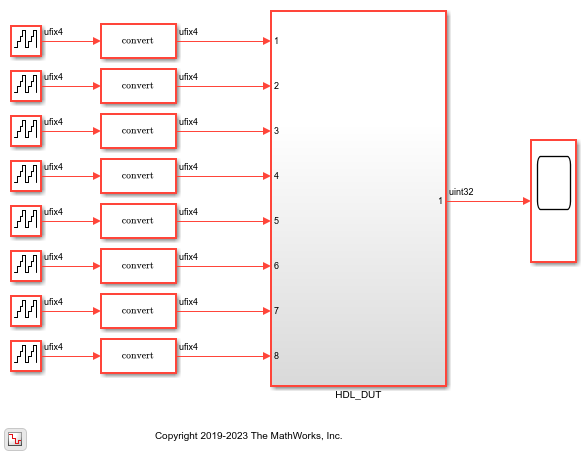# Distributed Pipeline Insertion for MATLAB Function Blocks

This example shows how to optimize the generated HDL code for MATLAB Function blocks by using the distributed pipelining optimization. Distributed pipelining is an HDL Coder™ optimization that improves the generated HDL code from MATLAB Function blocks, Simulink® models, or Stateflow® charts. By using distributed pipelining, your design achieves higher clock rates on the FPGA device.

### Multiplier Chain Model

This example shows how to distribute pipeline registers in a simple model that chains five multiplications.

```open_system('hdlcoder_distpipe_multiplier_chain') set_param('hdlcoder_distpipe_multiplier_chain','SimulationCommand','Update') ```The `HDL_DUT` Subsystem is the DUT for which you want to generate HDL code. The subsystem drives a MATLAB Function block `mult_chain`.

```open_system('hdlcoder_distpipe_multiplier_chain/HDL_DUT') ```To see the chain of multiplications, open the MATLAB Function block.

```open_system('hdlcoder_distpipe_multiplier_chain/HDL_DUT/mult_chain') ```### Apply Distributed Pipelining Optimization

1. Specify generation of two pipeline stages for the MATLAB Function block.

```ml_subsys = 'hdlcoder_distpipe_multiplier_chain/HDL_DUT/mult_chain'; hdlset_param(ml_subsys, 'OutputPipeline', 2) ```

2. Specify the `MATLAB Datapath` architecture. This architecture treats the MATLAB Function block like a regular Subsystem. You can then apply various optimizations across the MATLAB Function blocks with other blocks in your Simulink® model.

```hdlset_param(ml_subsys, 'architecture', 'MATLAB Datapath'); ```

3. Enable the distributed pipelining optimization on the block. To see the results of the optimization, enable generation of the Optimization Report. To apply the optimization across hierarchies in your model, enable hierarchical distributed pipelining on the model and distributed pipelining on all subsystems.

```hdlset_param('hdlcoder_distpipe_multiplier_chain', ... 'HierarchicalDistPipe', 'on', 'OptimizationReport', 'on') hdlset_param('hdlcoder_distpipe_multiplier_chain/HDL_DUT', 'DistributedPipelining', 'on'); hdlset_param(ml_subsys, 'DistributedPipelining', 'on'); ```

4. Generate HDL Code for the `HDL_DUT` subsystem.

```makehdl('hdlcoder_distpipe_multiplier_chain/HDL_DUT/mult_chain') ```

By default, HDL Coder generates VHDL code in the `hdlsrc` folder.

### Analyze Results of Optimization

In the Distributed Pipelining report, you see that the code generator moved the pipeline registers. To see the effects of the optimization, open the generated model `gm_hdlcoder_distpipe_multiplier_chain` and navigate to the `HDL_DUT` Subsystem.The `MATLAB Datapath` architecture creates a Subsystem in place of the MATLAB Function block. The optimization can then distribute the pipeline registers and the unit delay that you added inside the Subsystem to optimize the multiplier chain and improve timing. Open the `mult_chain` Subsystem.